Skip to main content

# Variable packet splitting transmission in multi-relay cooperative communications with DF and DAF for SC-FDMA

## Abstract

In recent years, cooperative communications are widely studied. Cooperative communications can obtain the space diversity as multiple-input multiple-output (MIMO) systems. In cooperative communications, the relay method is important as decode-and-forward (DF) and decode-amplify-forward (DAF). The multi-relay cooperative communications can improve the system performance. In the multi-relay cooperative communications, the optimum packet splitting method is effective. Moreover, the multi-relay cooperative communications can more improve the system performance by using the power allocation (PA). However, the PA method requires large feedback information (FBI). To solve this problem, in this article, we propose the optimum packet splitting method based on the time domain channel state information (CSI) in the multi-relay cooperative communication with DF and DAF.

## Introduction

As the next generation standard of the mobile communications, a long-term evolution (LTE)-Advanced is standardized . LTE-Advanced supports broadband data services with the maximum transmission rate of about 0.1–1 Gbps. In a LTE-Advanced, single carrier frequency division multiple access (SC-FDMA) has been adopt in the uplink communication, and orthogonal frequency division multiple access (OFDMA) has been adopt in the downlink communication . SC-FDMA can achieve a low peak average power ratio (PAPR) compared with OFDMA . Since a low PAPR of SC-FDMA can reduce the cost and the power consumption of communication, SC-FDMA is suitable transmission method for the uplink communication.

In recent years, cooperative communications are widely studied . Cooperative communications use the relay node except the source and destination nodes. By using the relay node, cooperative communications can obtain the space diversity as multiple-input multiple-output (MIMO) systems. For the problem of PAPR, the signals of each antenna have to process in the same device for MIMO systems. As a result, MIMO systems increase PAPR. On the other hand, the signals process in the independent device as the relay node for cooperative communications. Therefore, cooperative communications mitigate the problem of PAPR compared with MIMO systems [10, 11]. In cooperative communications, the relay method is important as amplify-and-forward (AF), decode-and-forward (DF), and decode-amplify-forward (DAF) . AF is the simplest method. This is because it is only adjust the amplitude of the signal in the relay node . However, since AF is poor in a frequency selective fading environment, the system performance is significantly degraded. To solve this problem, DF and DAF have been proposed . DF and DAF detect the received signal in the relay node. As a result, DF and DAF are strong in a frequency selective fading environment. For the deference of DF and DAF, DF detects the received signal by using the hard decision [15, 16]. On the other hand, DAF detects the received signal by using the soft decision . In this article, we use DF and DAF, and compare their performances.

In cooperative communications, the multi-relay cooperative communications have been proposed . This system can improve the system performance to obtain more strong diversity by using several relay nodes. However, if the channel condition of relays is bad, the system performance is degraded. To solve this problem, the optimum packet splitting has been proposed in MIMO systems . The optimum packet splitting method splits the packet by considering the channel condition and sends from each transmit antenna. As a result, the optimum packet splitting method can improve the system performance. However, the optimum packet splitting is not considered in the multi-relay cooperative communications. Therefore, in this article, we propose the optimum packet splitting in the multi-relay cooperative communication systems. Cooperative communications can improve the system performance by using the power allocation (PA) . This method is also effective in the multi-relay cooperative communications . Therefore,  has adopt the waterfilling power allocation (WF/PA) method. The WF/PA method mitigates the deep faded channel by using the optimum power . However, the PA method requires large feedback information (FBI) since it has to feedback the channel state information (CSI) of each sampling point in a frequency domain. To solve these problems, in this article, we propose the optimum packet splitting method based on the time domain CSI in the multi-relay cooperative communication with DF and DAF.

Common notations that E|·|, (·) denote the ensemble average operation, a complex conjugate, respectively.

## System model

In this article, we assume the multi-relay cooperative communications as shown in Figure 1. Here, we define the notation as follows.

• SD is the link between the source and destination nodes.

• SR is the link between the source and relay nodes.

• RD is the link between the relay and destination nodes.

• SRD is the link between the source, relay, and destination nodes.Figure 1

### Channel model

We assume that a propagation channel consists of L discrete paths with different time delays. The impulse response h n (τ,t) for the n th node is represented as

$h n (τ,t)= ∑ l = 0 L − 1 h n , l (t)δ(τ− τ n , l ),$
(1)

where hn,l, τn,l are the complex channel gain and the time delay of the l th propagation path for the n th node, and $∑ l = 0 L − 1 E| h n , l 2 |=1$, respectively. The channel transfer function H n (f,t) is the Fourier transform of h n (τ,t), and is given by

$H n ( f , t ) = ∫ 0 ∞ h n ( τ , t ) exp ( − j 2 Πfτ ) dτ = ∑ l = 0 L − 1 h n , l ( t ) exp ( − j 2 Πf τ n , l ) .$
(2)

### Source node

The source node block diagram of the proposed system is shown in Figure 2a. Firstly, the coded data is modulated, and is performed with interleaving. After the N c points IFFT operation, the time domain transmitted signal for the n th node s n (t) can be expressed as

$s n (t)= 2 E s T s ∑ k = 0 N c − 1 u n (k)exp j 2 Πk t N c ,$
(3)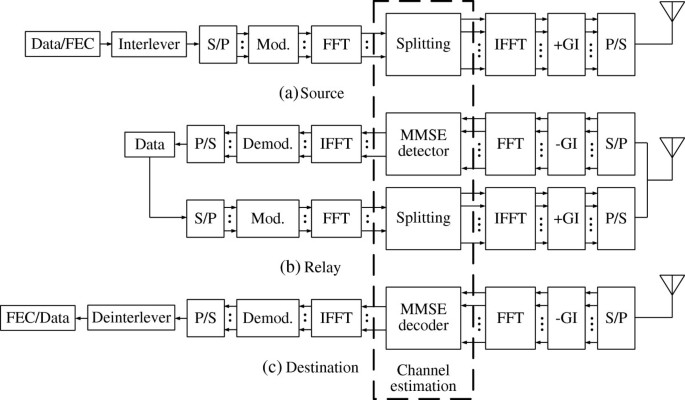Figure 2

where N c is the number of sampling points, T s is the effective symbol length, and E s is the average transmission power, respectively. For Equation (3), by using the N c points FFT operation, the frequency domain signal u n (k) is given by

$u n (k)= 2 E s N c ∑ t = 0 N c − 1 d n (t)exp − j 2 Πk t N c ,$
(4)

where d n (t) is the original signal for the n th node. From Equation (3), the proposed method splits the packet as shown in Figure 3. Here, the number of total data symbols N d is defined as

$N d = N D + ∑ i = 0 N − 1 N i , R ,$
(5)Figure 3

where N d is the number of data symbols for SD, Ni,Ris the number of data symbols for i th SRD, and N is the number of relay nodes, respectively. In this article, since we assume the multi-relay cooperative communications, we define N D ≥1 and Ni,R≥1. For the proposed packet splitting method, we explain in the next section. In general, the guard interval (GI) is inserted in order to eliminate the inter-symbol interference (ISI) due to a multipath fading, and hence, we have

$T= T s + T g ,$
(6)

where T g is the GI length.

### Relay node

The relay node structure is illustrated in Figure 2b. The received signal for the n th relay node $r ̂ n (t)$ can be expressed as

$r ̂ n (t)= ∫ − ∞ ∞ h n , sr (τ,t) s n (t−τ)dτ+ n n (t),$
(7)

where n n (t) is additive white Gaussian noise (AWGN) with a single sided power spectral density of N0. After removing the GI, by using the N c points FFT operation, Equation (7) is rewritten as

$r ̂ n (k)= 2 E s T s H n , sr (k) u n (k)+ n ̂ n (k),$
(8)

where $n ̂ n (k)$ is AWGN noise with zero-mean and a variance of 2N0/T s . By using the minimum mean square error (MMSE) equalization, the detected signal of Equation (8) $û n (k)$ is given by

$û n (k)= r ̂ n (k)· ω n , sr (k),$
(9)

where ωn,s r(k) is the MMSE weight. After the detection, the detected signal $û n (k)$ is also modulated and transmits to the destination node by using decode-and-forward (DF) or decode-amplify-forward (DAF).

### Destination node

The destination node structure is illustrated in Figure 2c. The received signal r(t) can be expressed as

$r ( t ) = r 0 ( t ) + ∑ n = 0 N − 1 r n ( t ) + n ( t ) = ∑ n = 0 N ∫ − ∞ ∞ h n ( τ , t ) s n ( t − τ ) dτ + n ( t ) ,$
(10)

where r0(t) is the received signal for SD, r n (t) is the n th received signal for RD, and n(t) is AWGN, respectively. After removing the GI, by using the N c points FFT operation, Equation (10) is rewritten as

$r ~ (k)= ∑ n = 0 N 2 E s T s H n (k) u n (k)+ n ̂ (k),$
(11)

where $n ̂ (k)$ is AWGN noise. By using the MMSE equalization, the detected signal of Equation (11) $ũ n (k)$ is given by

$ũ n (k)= r ( k ) · ω sd ( k ) for SD r ( k ) · ω n , rd ( k ) for RD ,$
(12)

where ω s d (k) and ωn,r d(k) are the MMSE weights for SD and n th RD, respectively. Finally, by using the N c points IFFT operation, the desired signal $d ~ n (t)$ is given by

$d ~ n (t)= 2 E s N c ∑ k = 0 N c − 1 ũ n (k)exp j 2 Π k t N c .$
(13)

### MMSE equalization

In this article, we assume the perfect channel estimation. The MMSE weight ω n (k) is given by

$ω n (k)= H n ∗ ( k ) | H n ( k ) | 2 + σ n 2 ( k ) ,$
(14)

where $σ n 2$ is the noise power .

## Proposed variable splitting method

In this section, we explain the waterfilling power allocation (WF/PA) method and the proposed variable splitting method.

### Waterfilling power allocation (WF/PA)

The waterfilling power allocation (WF/PA) method has been proposed to improve the system performance . The WF/PA method mitigates the deep faded channel by using the optimum power assignment. The optimum power of the k th sampling point for the n th node P n (k) is given by

$P n (k)= μ n − 1 | H n ( k ) | 2 for μ n ≥ 1 | H n ( k ) | 2 1 otherwise ,$
(15)

where the cutoff SNR revel μ n is

$μ n = 1 N c ∑ k = 0 N c − 1 1 | H n ( k ) | 2 .$
(16)

From Equation (15), the WF/PA method can improve the system performance. However, the WF/PA method requires large feedback information (FBI). To mitigate this problem, we propose the variable splitting method.

### Proposed variable splitting method

The proposed variable splitting method is performed based on the time domain CSI. For the time domain CSI, the amplitudes are constant. From this characteristic, since FBI of the proposed method becomes only one, the proposed method can reduce FBI compared with the WF/PA method.

Next, we explain the proposed method. The output signal X due to a Rayleigh fading is expressed as

$X= X c +j X s ,$
(17)

where X c is a real part and X s is a imaginary part, respectively . From Equation (17), the amplitude of X is given by

$|X|= X c 2 + X s 2 .$
(18)

By using Equation (18), the proposed method is performed. First, we define the amplitude for each propagation. The amplitude for SD (λ D ) is expressed as

$λ D =| X SD |,$
(19)

where X S D is the output signal due to a Rayleigh fading for SD. Also, the amplitude for the i th SRD (λi,R) is expressed as

$λ i , R = ( 1 − α ) | X i , SR | 2 + α | X i , RD | 2 ,$
(20)

where Xi,S Rand Xi,R Dare the output signals due to a Rayleigh fading for i th SR and i th RD, and α is the selection parameter for SRD, respectively. Then, we decide the number of data symbols for SD (N d ) and the number of data symbols for i th SRD (Ni,R). Firstly, the proposed method decides the number of data symbols for SD (N d ) as

$N D = N d × ∑ j = 0 N − 1 γ λ D λ D + λ j , R ,$
(21)

where γ is the selection parameter for SD and SRD, and x stands for the integer and closer to x, respectively. For the parameters α and γ, we decide them by using the computer simulation for DF and DAF in the next section. After choosing N d , the proposed method decides the number of data symbols for i th SRD (Ni,R). The number of data symbols for i th SRD (Ni,R) is given by

$N i , R =( N d − N D )× ∑ j = 0 N − 1 λ i , R λ j , R .$
(22)

By using Equations (21) and (22), the proposed method can achieve the optimum packet splitting.

## Computer simulation results

In this section, we show the performance of the proposed method. In our simulation, we have used MATLAB simulation. For increasing the simulation accuracy, we consider the Monte Carlo method for computer simulation. Figure 2 shows a simulation model of the proposed system. On the source node, the data stream is encoded. Here, convolutional codes (rate R = 1/2, constrain length $K=7$) with interleaving are used. The coded bits are QPSK modulated. After serial to parallel (S/P) converted, the FFT spreading operation is performed. By using the IFFT operation, the SC-FDM time domain signal is generated and is inserted a guard interval (GI). The SC-FDM time domain signal is split based on Equations (21) and (22), and is transmitted to the relay and destination nodes. In this simulation, we assume that the channel estimation is the perfect. Moreover, the SC-FDM symbol period is 2 μ s, the GI length is 125 ns, the path separation is Tpath = 37 ns, and the maximum Doppler frequency is 5 Hz. In the relay node, the received signal is detected as Equation (9). After the detection, the detected signal is modulated again, and is transmitted to the destination node. For the relay method, in this article, we assume the decoded-and-forward (DF) and the decode-amplify-forward (DAF). In the destination node, all received signals are combined, and are S/P converted. After removing the GI, the parallel sequences are passed to the FFT operator and convert the signal back to the frequency domain. The frequency domain data signal is detected by using the MMSE equalization. After the detection, the detected signal is converted to the time domain signal by the IFFT operation. And then, the total bits are decoded by using the Viterbi soft decoding algorithm. The packet consists of N d = 80 data symbols. Table 1 shows the simulation parameters.

First, we decide the optimum value of the parameters α and γ for DF. Figure 4 shows the BER versus the parameter α for γ = 0.1, 1, 2, 3, and 4 with E b /N0 = 20 dB at Doppler frequency of 5 Hz. From the simulation result, when α is small and γ is large, the proposed method shows the good BER performance. Moreover, when α = 0.1 and γ = 4, the proposed method shows the best BER performance. Figure 5 shows the BER versus the parameter γ for α = 0, 0.1, 0.2, 0.3, and 0.4 with E b /N0 = 20 dB at Doppler frequency of 5 Hz. From the simulation result, when α is small and γ is large, the proposed method shows the good BER performance. Moreover, when α = 0.1 and γ = 4, the proposed method shows the best BER performance as same Figure 4. On the other hand, when γ is greater than 4, the proposed method shows the bad BER performance. From Figures 4 and 5, we decide the parameters as α = 0.1 and γ = 4 for DF.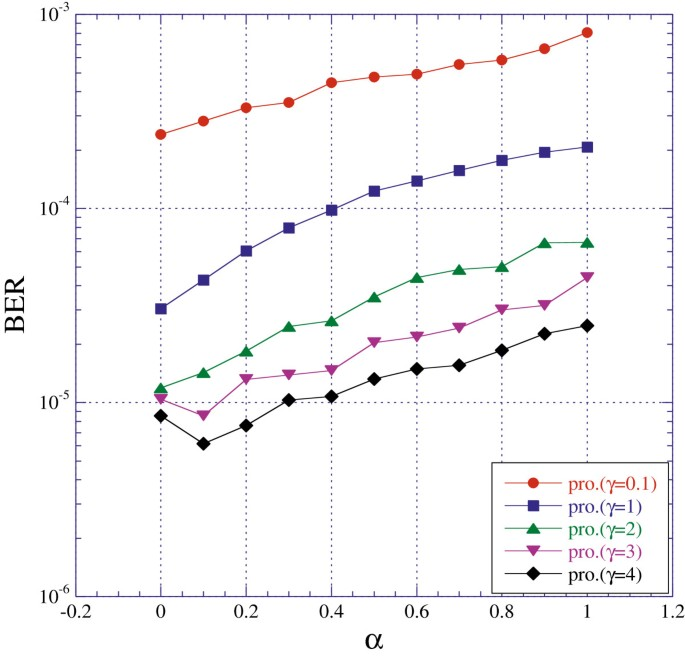Figure 4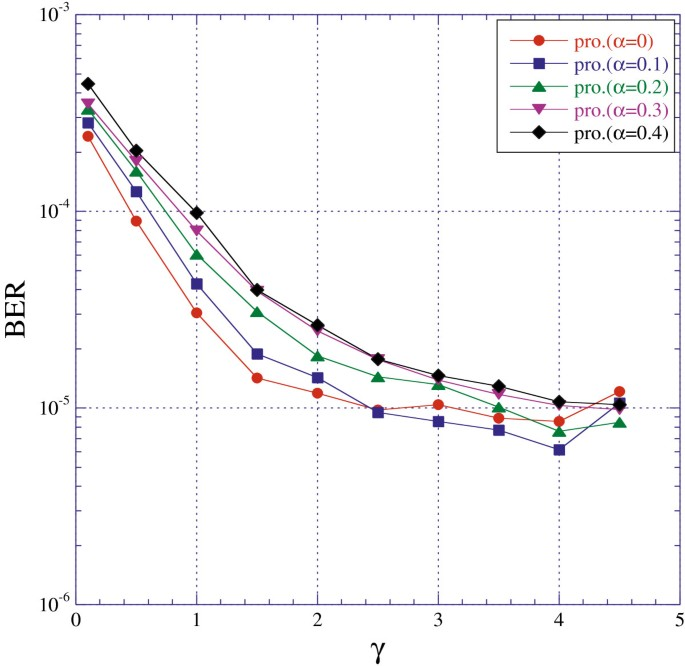Figure 5

Next, we decide the optimum value of the parameters α and γ for DAF. Figure 6 shows the BER versus the parameter α for γ = 0.1, 1, 2, 3, and 4 with E b /N0 = 20 dB at Doppler frequency of 5 Hz. From the simulation result, when α is small and γ is large, the proposed method shows the good BER performance. Moreover, when α = 0.4 and γ = 3, the proposed method shows the best BER performance. Figure 7 shows the BER versus the parameter γ for α = 0, 0.1, 0.2, 0.3, and 0.4 with E b /N0 = 20 dB at Doppler frequency of 5 Hz. From the simulation result, when α is small and γ is large, the proposed method shows the good BER performance as same Figure 5. Moreover, when α = 0.4 and γ = 3.5, the proposed method shows the best BER performance. On the other hand, when γ is greater than 3.5, the proposed method shows the bad BER performance. From Figures 6 and 7, we decide the parameters as α = 0.4 and γ = 3.5 for DAF.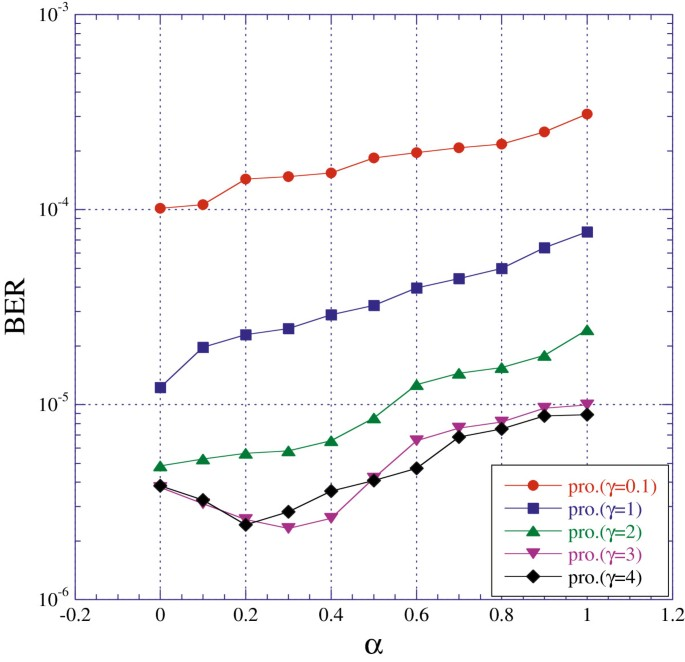Figure 6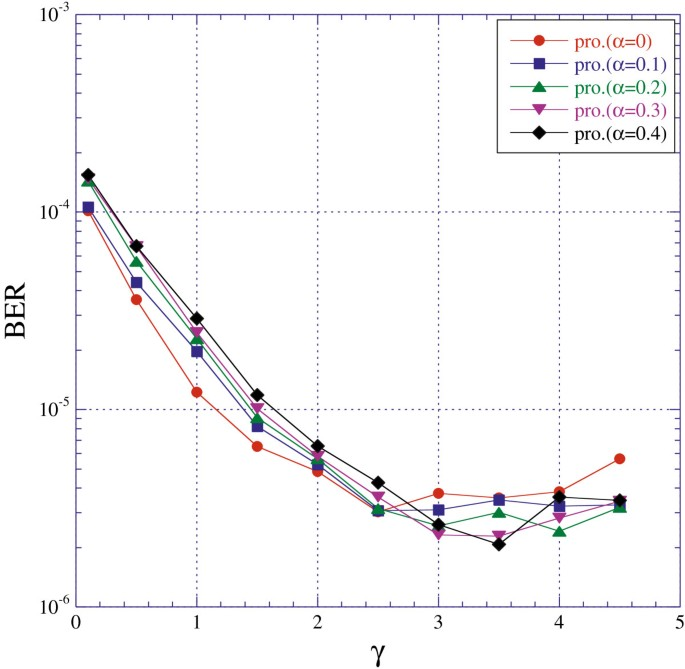Figure 7

Finally, we show the BER performance of the proposed method. Figure 8 shows the BER of the conventional and proposed methods at Doppler frequency of 5 Hz. First, the BER of the conventional method N D = 40 and Ni,R= 20 shows about 4 dB gain compared with N = 0, where i = 1,2. This is because the conventional method of N D = 40 and Ni,R= 20 obtains the strong space diversity by using two relay nodes. Next, the BER of WF/PA shows about 2 dB gain compared with N D = 40 and Ni,R= 20. This is because the WF/PA method mitigates the deep faded channel by using the optimum power assignment. However, the WF/PA method requires large FBI. The proposed method reduces FBI compared with the WF/PA method. Moreover, the BER of the proposed method shows about 2 dB gain compared with the WF/PA method. This is because the proposed method obtains the space diversity and coding gains by using the optimum packet splitting. Finally, we compare the BER performance for DF and DAF. For the conventional and proposed methods, the BER of DAF shows the good BER performance compared with DF. This is because the signal with DAF can obtain the more accurate detected signal compared with DF in the relay node. Moreover, the BER of the proposed method for DAF shows about 1.5 dB gain compared with DF. Therefore, the proposed method for DAF is effective, and shows the best BER performance.Figure 8

## Conclusion

In this article, we have proposed the variable packet splitting method in the multi-relay cooperative communications with DF and DAF. The proposed method obtains the space diversity by using the multi-relay cooperative communications. Moreover, since the proposed method uses the error correcting code and interleaving, obtains also the coding gain. However, if the channel condition of relays is bad, the system performance is degraded. Therefore, the proposed method splits the packet to obtain the space and coding gains. Moreover, the proposed method uses the selection parameters α and γ to obtain the maximum gains. As a result, the proposed method obtains the maximum coding gain due to the space diversity. From the simulation results, the proposed method has decided the optimum value of selection parameters α and γ, and achieved the best BER performance. Moreover, the proposed method of DAF has shown the good BER performance compared with DF.

## References

1. 1.

Abeta S: Toward LTE commerical launch and future plan for LTE enhancements (LTE-Advanced). In Proc. of 12th IEEE International Conference on Communication Systems (ICCS). Singapore; 2010:146-150.

2. 2.

Noune M, Nix A: Frequency-domain precoding for single carrier frequency-division multiple access. IEEE Commun. Mag 2009, 47(6):68-74.

3. 3.

Adachi F, Tomeba H, Takeda K: Frequency-domain equalization for broadband single-carrier multiple access. IEICE Trans. Commun 2009, E92-B(5):1441-1456. 10.1587/transcom.E92.B.1441

4. 4.

Luo X, Gaal P, Chen W, Zhang X, Montojo J: Transmit diversity scheme over single SC-FDM symbol for LTE-Advanced. In Proc. of 2009 IEEE GLOBAL COMMUNICATIONS CONFERENCE (GLOBECOM). Hawaii, USA: Honolulu; 2009:1-6.

5. 5.

Zhang C, Wang Z, Yang Z, Wang J, Song J: Frequency domain decision feedback equalization for uplink SC-FDMA. IEEE Trans. Broadcast 2010, 56(2):253-257.

6. 6.

Laneman JN, Tse DNC, Wornell GW: Cooperative diversity in wireless networks: efficient protocols and outage behavior. IEEE Trans. Inf. Theory 2004, 50(12):3062-3080. 10.1109/TIT.2004.838089

7. 7.

Florea AA, Gacanin H, Adachi F: Performance comparison of cooperative OFDM and SC-FDE relay networks in a frequency-selective fading channel. In Proc. of 12th IEEE International Conference on Communication Systems (ICCS). : Singapore; 2010:371-375.

8. 8.

Eghbali H, Muhaidat S, Dhahir NA: A new receiver design for single-carrier frequency domain equalization in broadband cooperative wireless networks. In Proc. of 2009 IEEE GLOBAL COMMUNICATIONS CONFERENCE (GLOBECOM). Florida, USA: Miami; 2010:1-6.

9. 9.

Zhang J, Yang L, Hanzo L: Energe-efficient channel-dependent cooperative relaying for the multiuser SC-FDMA uplink. IEEE Trans. Veh. Tech 2011, 60(3):992-1004.

10. 10.

Kim Y-J, Kwon U-K, Seol D-Y, Im G-H: An effective PAPR reduction of SFBC-OFDM for multimode cooperative transmission. IEEE Signal Process. Lett 2009, 16(11):925-928.

11. 11.

Eddaghel MM, Chambers JA: PAPR reduction in distributed amplify-and-forward type closed loop extended orthogonal space frequency block coding with one-bit group feedback for cooperative communications. In Proc. of 18th European Wireless Conference (EW). Poland: Poznan; 2012:1-6.

12. 12.

Zhao Y, Adve R, Lim TJ: Improving amplify-and-forward relay networks: optimal power allocation versus selection. IEEE Trans. Wirel. Commun 2007, 6(8):3114-3123.

13. 13.

Kaneko M, Hayashi K, Popovski P, Ikeda K, Sakai H, Prasad R: Amplify-and-forward cooperative diversity schemes for multi-carrier systems. IEEE Trans. Wirel. Commun 2008, 7(5):1845-1850.

14. 14.

Liang Y, Schober R: Cooperative amplify-and-forward beamforming for OFDM systems with multiple relays. In Proc. of 2009 IEEE International Confernece on Communications (ICC). Germany: Dresden; 2009:1-6.

15. 15.

Wang J, Liang J, Muhaidat S: Distortion exponents for decode-and-forward multi-relay cooperative networks. In Proc. of 2009 IEEE International Symposium on Information Theory (ISIT). Korea: Seoul; 2009:854-858.

16. 16.

Lee Y, Chen YN, Sou SI: Error-rate performance of uplink cooperative communications with decode-and-forward protocol. IET Commun 2011, 5(11):1484-1490. 10.1049/iet-com.2010.0270

17. 17.

Bao X, Li J: Efficient message relaying for wireless user cooperation: decode-amplify-forward (DAF) and hybrid DAF and coded-cooperation. IEEE Trans. Wirel. Commun 2007, 6(11):3975-3984.

18. 18.

Fukuyama Y, Takyu O, Adachi K, Nakagawa M: Relay method of sending soft decision symbol based on the result of error detecting code in cooperative communication. IEICE Trans. Fundament 2007, E90-A(11):2404-2412. 10.1093/ietfec/e90-a.11.2404

19. 19.

Duong TQ, Zepernick HJ: Hybrid decode-amplify-forward cooperative communications with multiple relays. In Proc. of 2009 IEEE Wireless Communications and Networking Conference (WCNC). Hungary: Budapest; 2009:1-6.

20. 20.

Olfat E, Olfat A: Performance of hybrid decode-amplify forward protocol for multiple relay networks over independent and non-identical flat fading channels. IET Commun 2011, 5(14):2018-2027. 10.1049/iet-com.2010.0834

21. 21.

Song K, Kim J, No J, Chung H: Analysis on soft-decision-and-forward cooperative networks with multiple relays. IEICE Trans. Commun 2012, E95-B(2):509-518. 10.1587/transcom.E95.B.509

22. 22.

Chen S, Wang W, Zhang X: Performance analysis of multiuser diversity in cooperative multi-relay networks under rayleigh-fading channels. IEEE Trans. Wirel. Commun 2009, 8(7):3415-3419.

23. 23.

Sun S, Hunziker T, Cheng J, Taromaru M, Ohira T: Optimal frame splitting for downlink MIMO channels with distributed antenna arrays. IEEE Trans. Veh. Tech 2008, 57(6):3848-3854.

24. 24.

Noune M, Nix A: Performance of SC-FDMA with transmit power allocation and frequency-domain equalization. In Proc. of 21st IEEE International Symposium on Personal, Indoor and Mobile Radio Communications (PIMRC). Turkey: Istanbul; 2010:852-857.

25. 25.

Lozano A, Tulino AM, Verdu S: Multiuser merculy/waterfilling for downlink OFDM with arbitrary signal constellations. In Proc. of 9th IEEE International Symposium on Spread Spectrum Techniques and Applications (ISSSTA). Amazon, Brazil: Manaus; 2006:292-296.

26. 26.

Wang B, Zhao D: Optimum power distributed for uplink channel in a cooperative wireless CDMA network. In Proc. of 2008 IEEE International Confernece on Communications (ICC). China: Beijing; 2008:4795-4801.

27. 27.

Ida Y, Ahn C, Kamio T, Fujisaka H, Haeiwa K, Omori T, Hashimoto K: Optimum splitting transmission in multi-relay cooperative communications for WF/PA-SC-FDMA. IEICE Tech. Rep., CS2011-127 2012, 157-160.

28. 28.

Li Y, Vucetic B, Zhou Z, Dohler M: Distributed adaptive power allocation for wireless relay networks. IEEE Trans. Wirel. Commun 2007, 6(3):948-958.

29. 29.

Ida Y, Ahn C, Kamio T, Fujisaka H, Haeiwa K: Throughput performance enhancement for MUDiv/OFDMA using MMSE equalization without guard interval. J. Sel. Area Telecommun 2011, 2(5):74-80.

30. 30.

Xiao C, Zheng YR, Beaulieu C: Statistical simulation models for rayleigh and rician fading. In Proc. of 2003 IEEE International Confernece on Communications (ICC). Alaska, USA: Anchorage; 2003:3524-3529.

Download references

## Author information

Correspondence to Yuta Ida.

## Additional information

### Competing interests

The authors declare that they have no competing interests.

## Authors’ original submitted files for images

Below are the links to the authors’ original submitted files for images.

## Rights and permissions

Open Access This article is distributed under the terms of the Creative Commons Attribution 2.0 International License (https://creativecommons.org/licenses/by/2.0), which permits unrestricted use, distribution, and reproduction in any medium, provided the original work is properly cited.

Reprints and Permissions

## About this article

### Keywords

• SC-FDMA
• Cooperative communications
• Multi-relay
• Decode-and-forward
• Decode-amplify-forward
• Variable packet splitting